## RS Aggarwal Class 8 Solutions Chapter 17 Construction of Quadrilaterals Ex 17B

These Solutions are part of RS Aggarwal Solutions Class 8. Here we have given RS Aggarwal Solutions Class 8 Chapter 17 Construction of Quadrilaterals Ex 17B.

Other Exercises

Question 1.
Solution:
Steps of Construction :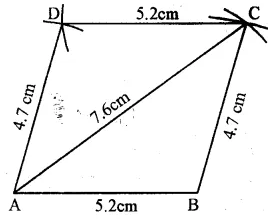(i) Draw a line segment AB = 5.2 cm.
(ii) With centre A and radius 7.6 cm. and with centre B and radius 4.7 cm. draw arcs which intersect each other at C.
(iii) Join AC and BC.
(iv) Again with centre A and radius 4.7 cm and with centre C and radius 5.2 cm draw arcs which intersect each other at D.
ABCD is the required parallelogram.

Question 2.
Solution:
Steps of Construction :
(i) Draw a line segment AB = 4.3 cm.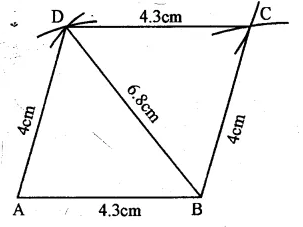(ii) With centre A and radius 4 cm. and with centre B and radius 6.8 cm., draw arcs which intersect each other at D.
(iv) Again with centre B and radius 4 cm. and with centre D and radius 4.3 cm., draw arcs intersecting each other at C.
(v) Join DC and BC. ABCD is the required parallelogram.

Question 3.
Solution:
Steps of Construction :
(i) Draw a line segment PQ = 4 cm.
(ii) At Q, draw a ray making an angle of 60° and cut off QR = 6 cm.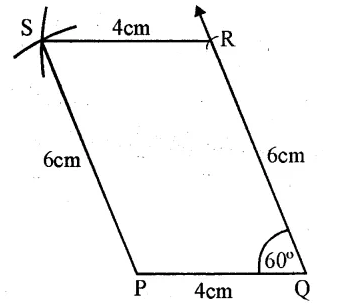(iii) With centre P and radius 6 cm. and with centre R and radius 4 cm draw arcs intersecting each other at S.
(iv) Join RS and PS.
PQRS is the required parallelogram. Q.

Question 4.
Solution:
Steps of Construction :
(i) Draw a line segment BC = 5 cm.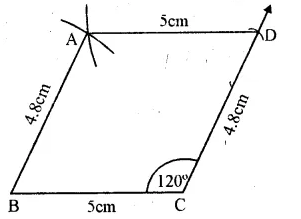(ii) At C, draw a ray making an angle of 120° and cut off CD = 4.8 cm.
(iii) With centre B and radius 4.8 cm. with centre D and radius 5 cm, draw arcs intersecting each other at A.
ABCD is the required parallelogram.

Question 5.
Solution:
Steps of Construction :
We know that diagonals of a parallelogram bisect each other.
(i) Draw a line segment AB = 4.4 cm.
(ii) With centre A and radius $$\\ \frac { 5.6 }{ 2 }$$ cm and with centre B and radius $$\\ \frac { 7 }{ 2 }$$ = 3.5 cm. draw arcs
intersecting each other at O.
(iii) Join AO and BO and produce them to C and D respectively such that OC = 2.8 cm and OD = 3.5 cm.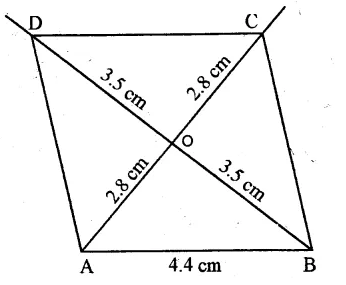(iv) Join AD, CD and BC
ABCD is the required parallelogram

Question 6.
Solution:
Steps of Construction :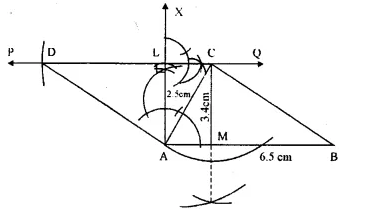(i) Draw a line segment AB = 6.5 cm.
(ii) At A, draw a perpendicular AX and cut off AL = 2.5 cm.
(iii) Through L, draw a line PQ parallel to AB.
(iv) From A, draw an arc of radius 3 -4 cm which intersects the line PQ at C.
(v) Join AC. BC
(vi) From PQ, cut off CD = AB.
(viii) From C, draw a perpendicular CM to AB.
ABCD is the required parallelogram.

Question 7.
Solution:
Steps of Construction :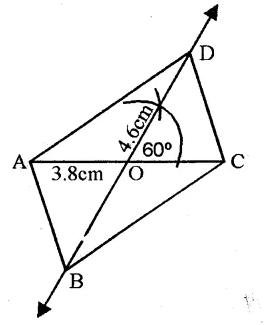(i) Draw a line segment AC = 3.8 cm.
(ii) Bisect it at O.
(iii) At O, draw a ray making an angle of 60° and produce it both sides.
(iv) From O cut off OB = OD = 2.3 cm.
(v) Join AB, BC, CD and AD.
ABCD is the required parallelogram.

Question 8.
Solution:
Steps of Construction :
(i) Draw a line segment AB = 11 cm.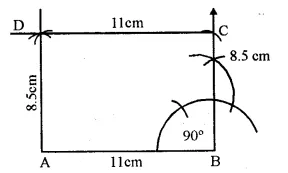(ii) At B, draw a perpendicular and cut off BC = 8.5 cm.
(iii) With centre A and radius 8.5 cm and with centre C and radius 11 cm, draw arcs intersecting each other at D.
ABCD is the required rectangle.

Question 9.
Solution:
Steps of Construction :
(i) Draw a line segment AB = 6.4 cm.(ii) At A and B draw perpendiculars and
cut off AD = BC = AB = 6.4 Cm.
(iii) Join CD.
ABCD is the required square.

Question 10.
Solution:
Steps of Construction :
(i) Draw a line segment AC = 5.8 cm.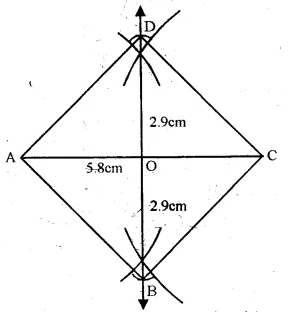(ii) Draw its perpendicular bisector intersecting AC at O.
(iii) From O, cut off OD = OB = 2.9 cm.
$$\qquad =\left( \frac { 1 }{ 2 } BD \right)$$
(iv) Join AB, BC, CD and DA. ABCD is the required square.

Question 11.
Solution:
Steps of Construction :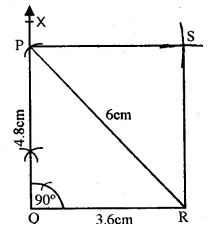(i) Draw a line segment QR = 3.6 cm.
(ii) At Q, draw a ray QX making an angle of 90°.
(iii) With centre R and radius 6 cm. draw an arc which intersects QX at P.
(iv) Join PR.
(v) With centre P and radius equal to QR and with centre R and radius equal to QP, draw arcs intersecting each other at S.
(vi) Join PS and RS.
PQRS is the required rectangle.
The length of other side PQ = 4.8 cm.

Question 12.
Solution:
Steps of Construction :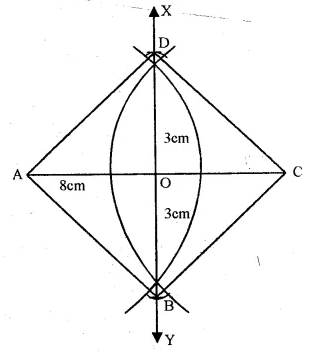(i)Draw a line segment AC = 8 cm.
(ii)Draw its perpendicular bisector intersecting it at O.
(iii)From O, cut off OB = OD = 3 cm.
(iv)Join AB, BC, CD and DA.
ABCD is the required rhombus.

Question 13.
Solution:
Steps of Construction :
(i)Draw a line segment AC = 6.5 cm.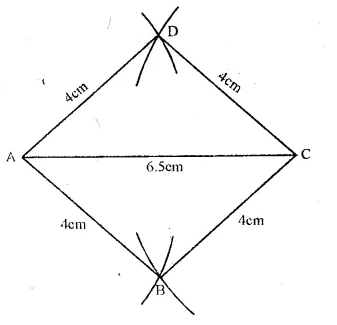(ii) With centres A and C and radius equal to 4 cm., draw arcs which intersect each other on both sides of line segment AC at B and D respectively.
(iii) Join AB, BC, CD and DA.
ABCD is the required rhombus.

Question 14.
Solution:
Steps of Construction :(i)Draw a line segmentAB = 7.2 cm.
(ii)At A draw a ray AX making an angle of 60° and cut off AD = 7.2 cm.
(iii)With centres D and B, and radius 7.2 cm., draw arcs intersecting each other at C.
(iv)Join CD and CB.
ABCD is the required rhombus.

Question 15.
Solution:
Steps of Construction :
(i) Draw a line segment AB = 6 cm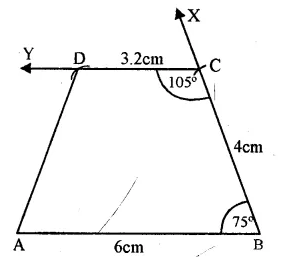(ii)At B, draw a ray BX making an angle of 75° and cut off BC = 4 cm.
(iii) At C, draw a ray CY making an angle of 180° – 75° = 105°
So that CY may be parallel to AB.
(iv) From CY, Cut off CD = 3.2 cm.
(v) Join DA.
ABCD is the required trapezium.

Question 16.
Solution:
Steps of Construction :
(i) Draw a line segment AB = 7 cm.(ii) At B, draw a ray BX making an angle of 60° and cut off BC = 5 cm.
(iii) At C, draw a ray CY making an angle of (180° – 60°) = 120° so that CY || AB.
(iv) With centre A and radius 6.5 cm. draw an arc intersecting CY at D.